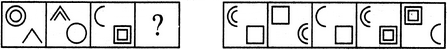# Non Verbal Reasoning - Analogy - Discussion

### Discussion :: Analogy - Section 1 (Q.No.4)

Each of the following questions consists of two sets of figures. Figures A, B, C and D constitute the Problem Set while figures 1, 2, 3, 4 and 5 constitute the Answer Set. There is a definite relationship between figures A and B. Establish a similar relationship between figures C and D by selecting a suitable figure from the Answer Set that would replace the question mark (?) in fig. (D).

4.

Select a suitable figure from the Answer Figures that would replace the question mark (?).(A)     (B)      (C)     (D)                  (1)      (2)      (3)      (4)      (5)

 [A]. 1 [B]. 2 [C]. 3 [D]. 4 [E]. 5

Explanation:

Each one of the upper elements is replaced by an element similar to the lower element(s) and each one of the lower elements is replaced by an element similar to the upper element(s).

 Sushil Jaiswal said: (Sep 23, 2018) I cannot understand this. Explain the solution.

 Aparna Mishra said: (Aug 14, 2020) I can't understand this please explain the solution.